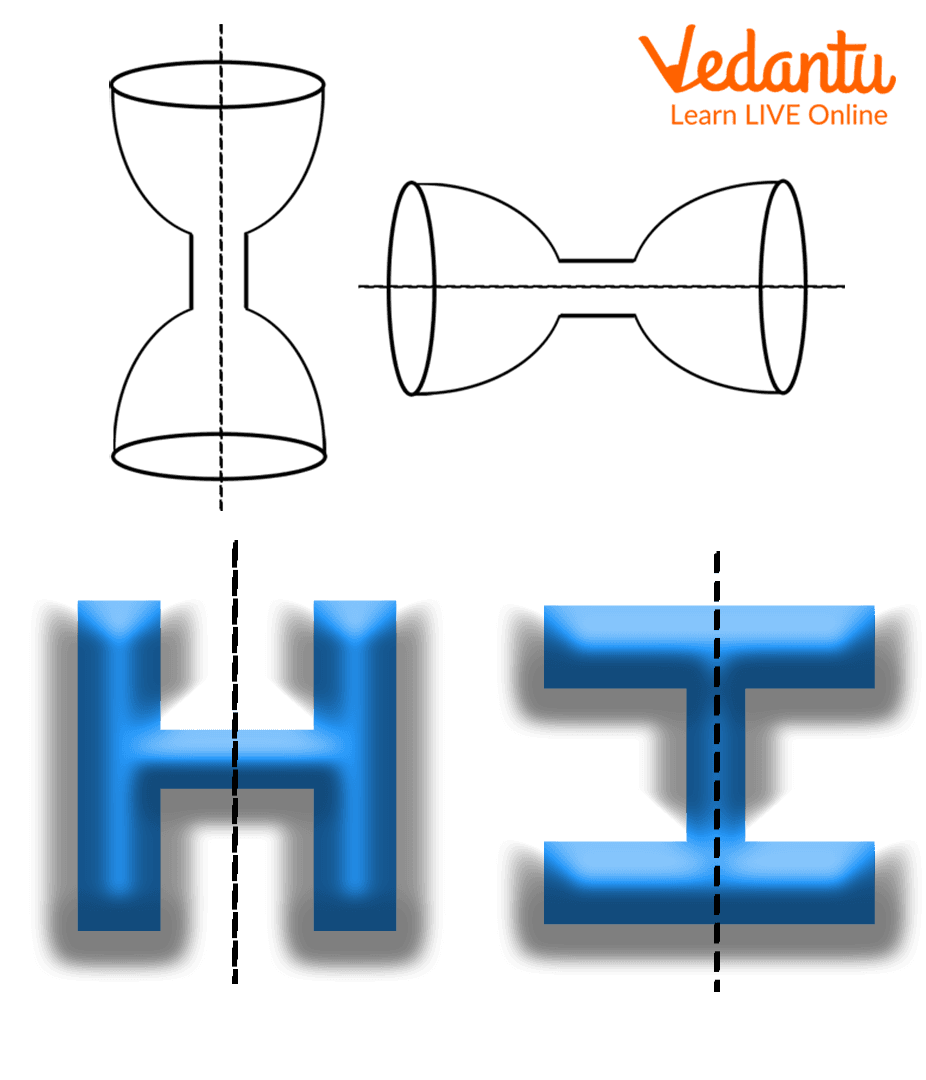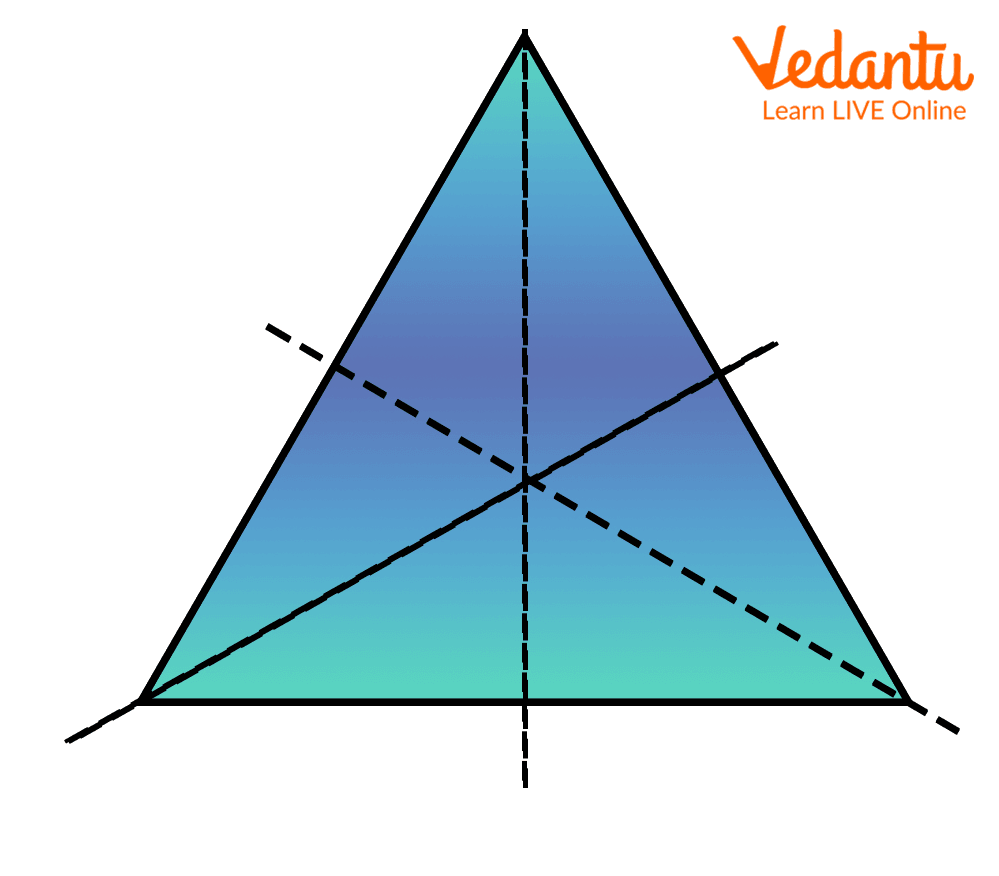Courses
Courses for Kids
Free study material
Free LIVE classes
More

# Symmetric Figures For KidsLIVE
Join Vedantu’s FREE Mastercalss

## What Is ‘Symmetric’?

What is your favourite snack? Or, what did you eat for breakfast? A sandwich.A Veg sandwich - Symmetric figures

Did your sandwich look the same as shown in the above figure? Probably your’s was filled with a different filling. But, here the point is to be focused on the shape of the sandwich. Both the parts of the sandwich are similar in looks and shape. And, if you put one part over another, then only the height will increase nothing else. Isn't it? So, both the parts are called symmetric.

## What Are Symmetric Figures?

If we cut an object into two parts such that both are equal in size and shape, then the obtained figures are called symmetric figures. If we put a mirror in front of a part then we can see the complete image of the object. The object and the reflection of an image are identical in shape and size. The line at which we cut an object to obtain symmetric images, is called the ‘line of symmetry’.

## How to Check If a Figure Is a ‘Symmetric Figure’ or Not?

To check whether the given figure or object is symmetric or not, we divide an object into two parts, keeping in mind that the division line passes from its centre. Then, we put one part over another. If there is no difference in the shape, then the figure is a ‘symmetric figure’, else it is called a ‘non-symmetric figure’ or asymmetrical figure.

## Line of Symmetry

The line of symmetry is the line that divides the object into two equal parts. An object can have one or more lines of symmetry. If an object or figure does not have any line of symmetry then it is called an asymmetric object or figure.

Here are some examples of symmetric figures with their line of symmetry:

1. Object of Only One Line Symmetry

If there is only one line by which we can divide an object into two equal parts, it is called an object of one line of symmetry. Some English alphabets that show the symmetry of one line are shown in the below figure.Examples of one line of symmetry

2. Object of Two Lines of Symmetry

If the object has two lines of symmetry i.e., there are two ways to fold a figure and obtain symmetric images, then the object is called an object of two lines of symmetry. A rectangle has two lines of symmetry.Examples of the figures shows two lines of symmetry

3. Object of Three Lines of Symmetry

An equilateral triangle has three lines of symmetry, these lines are along its three medians.Object of Three Lines of Symmetry

4. Object of Four Lines of Symmetry

Square has an object that has four lines of symmetry. These lines are vertical and horizontal axis passes by its centre, whereas two other lines of symmetry are its diagonals.Rectangle has two lines of symmetry

## Do You Know?

• A circle has an infinite number of lines of symmetry.

• A sunflower also shows symmetry.

• A kite that has four sides has only one line of symmetry.

Count the number of lines of symmetry in a sunflower, and ask your parents or teachers whether you are right or not.

## Conclusion

In this article, we have learnt about symmetric figures and lines of symmetry. A figure has one or more symmetry lines, but some figures that do not show any line of symmetry are known as asymmetrical.

Many geometrical figures have more than four lines of symmetry. Can you name them? Yes, a regular pentagon, regular hexagon and so on. If you enjoyed learning about this interesting Maths concept, visit our website to access more of such articles.

Last updated date: 23rd Sep 2023
Total views: 131.1k
Views today: 1.31k

## FAQs on Symmetric Figures For Kids

1. Define line of symmetry.

A line of symmetry is an imaginary line or axis along which if an object or figure folds or cuts then both the obtained parts are identical. It is also called the ‘axis of symmetry’ or ‘mirror line’ as it divides an object into two equal parts. A figure or object might have one or more than one line of symmetry whereas some objects have no line of symmetry. A figure is called asymmetrical if there is no line of symmetry for that object.

2. Name any three animals that show one line of symmetry.

Dog, rat and cat are examples of animals that show symmetry of one line. Also, humans show the symmetry of one line, along their vertical axis.